# Baric algebra

weighted algebra

In 1939, in connection with the formalism of genetics, I.M.H. Etherington introduced the notion of baric algebra (cf. [a1]; more commonly it is also called a weighted algebra). Ifis a (commutative) field anda-algebra, not necessarily commutative or associative, one says thatis a weighted algebra if there exists an algebra morphism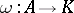which is non-trivial. This means that one can write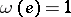, whereis a convenient element of. The morphismis called the weight function ofand one can regard a weighted algebra as a pair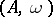whereis an algebra andthe weight function. A morphismof weighted algebras is a morphism of algebras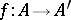such that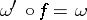. This gives a category, the category of weighted algebras. All constructions on weighted algebras are made in this category. For a finite-dimensional-algebra, the following conditions are equivalent:

i)is a weighted algebra;

ii) there exists a finite basisofoversuch that if(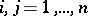) is the multiplication table ofin this basis (the scalars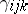(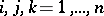) are the structure constants of), then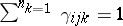();

iii) there exists a two-sided ideal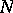of, of codimension one, such that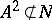.

It is easy to see that a Lie algebra is not weighted; however, over any weighted algebraone can define a Lie algebra structure via the multiplication (bracket)for all. A Jordan algebra may or may not be weighted; however, over any weighted algebra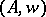one can define a Jordan algebra structure via the multiplication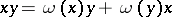for all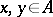. A weighted algebra is not necessarily associative (cf. Associative rings and algebras); however, over any weighted algebrawith a unitone can define an associative algebra structure via the multiplicationfor all. A Clifford algebra is not weighted; in particular, the algebra of complex numbers and the algebra of quaternions are not weighted. All Bernstein algebras are weighted (cf. Bernstein algebra).

How to Cite This Entry:
Baric algebra. Encyclopedia of Mathematics. URL: http://encyclopediaofmath.org/index.php?title=Baric_algebra&oldid=45325
This article was adapted from an original article by A. Micali (originator), which appeared in Encyclopedia of Mathematics - ISBN 1402006098. See original article